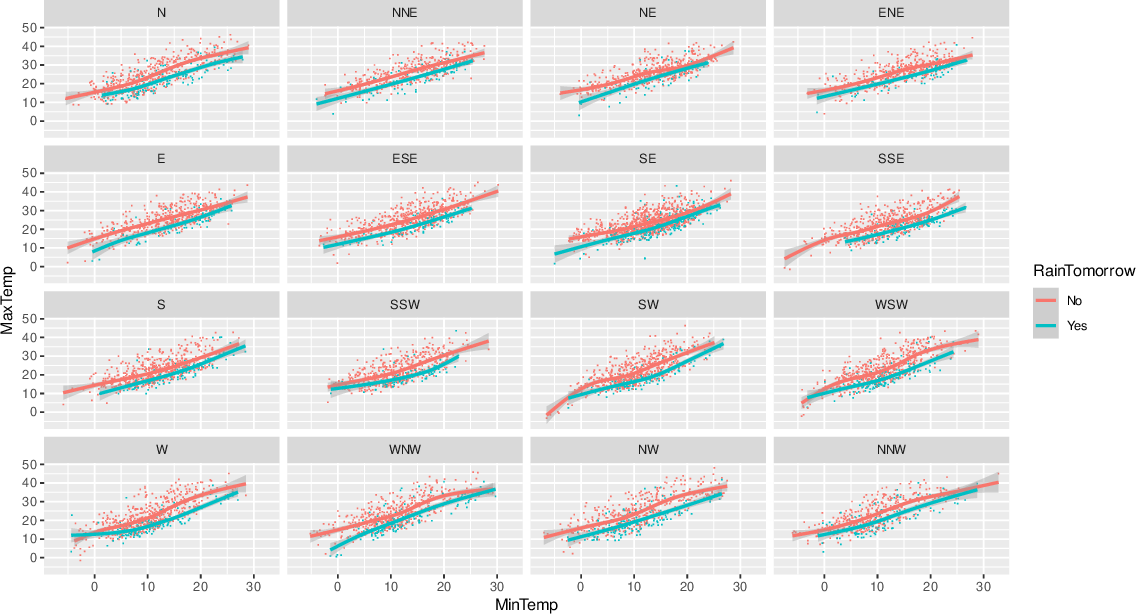Data Science Desktop Survival Guide by Graham WilliamsDesktop Survival Project Home Preface Data Science Introducing R R Constructs R Tasks R Strings R Read, Write, and Create Data Template Data Exploration Data Wrangling Data Visualisation Statistics ML Template ML Scenarios ML Activities ML Applications ML Algorithms Cluster Analysis Decision Trees Computer Vision Graph Data Privacy Literate Data Science Coding with Style Resources Bibliography Index

## Faceted Wind Direction

20200608lblr <- function(x) {   x %>%     str_replace_all("n", "North ") %>%     str_replace_all("s", "South ") %>%     str_replace_all("e", "East ") %>%     str_replace_all("w", "West ") %>%     str_replace(" \$", "") } ds %>%   sample_n(10000) %>%   ggplot(aes(x=min_temp, y=max_temp, colour=rain_tomorrow)) +   geom_point(shape=".") +   geom_smooth(method="gam", formula=y~s(x, bs="cs")) +   facet_wrap(~wind_dir_3pm, labeller=labeller(wind_dir_3pm=lblr)) +   labs(x      = vnames["min_temp"],        y      = vnames["max_temp"],        colour = vnames["rain_tomorrow"]) Labels of a faceted plot can be modified as here expanding n to North, s to South, etc. Observe that the linear relationship for rainy days is below that for dry days. The maximum temperature is generally closer to the minimum temperature on days where it rains the following day. The function to remap the directions uses stringr::str_replace_all() to do the work. It is then transformed into a ggplot2::labeller() for wind_dir_3pm=.